# How to write a diagonal matrix in LaTeX?

Elements of the diagonal matrix will be along the diagonal. All the other positions except the diagonal position of the matrix will be filled by zero.

\documentclass{article}
\usepackage{amsmath}
\begin{document}
$\begin{bmatrix} a_{1} & & & \\ & a_{2} & & \\ & & \ddots & \\ & & & a_{3} \end{bmatrix}$
\end{document}

Output :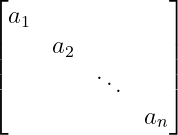And diagonal matrix is only valid in the case of a square matrix.

## Use physics package for diagonal matrix

In Latex, there are various arguments within the physics package that allow you to easily represent the diagonal matrix in a variety of ways.

\documentclass{article}
\usepackage{physics}
\begin{document}
$\mqty[ a_{1} & 0 & 0\\ 0 & a_{2} & 0\\ 0 & 0 & a_{3} ]$
\end{document}

Output :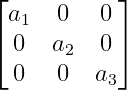Second, you can use the \dmat command. However, this \dmat command must be passed as an argument in the \mqty command without using direct.

\documentclass{article}
\usepackage{physics}
\begin{document}
$\mqty[\dmat{1,2,3,4}]$
\end{document}

Output :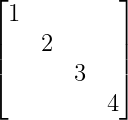And with that, the elements of the diagonal matrix have to be passed into \dmat command.

To fill in any position other than the diagonal position of the matrix by zero, you need to pass zero in the optional argument with \dmat command.

\documentclass{article}
\usepackage{physics}
\begin{document}
$\mqty[\dmat{1,2,3,4}]$
\end{document}

Output :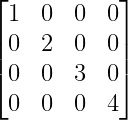## Inverse diagonal matrix in LaTeX

If you want to arrange the elements of the matrix along the inverse diagonal you need to use \admat command instead of \dmat command.

\documentclass{article}
\usepackage{physics}
\begin{document}
$\mqty[\admat{1,2,3,4}]$
$\mqty[\admat{1,2,3,4}]$
\end{document}

Output :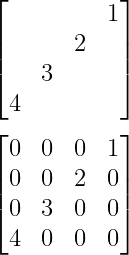#### Md Jidan Mondal

LaTeX expert with over 10 years of experience in document preparation and typesetting. Specializes in creating professional documents, reports, and presentations using LaTeX.